Chapter Notes: Introduction to Trigonometry

# Introduction to Trigonometry Chapter Notes - Mathematics (Maths) Class 10

 Table of contentsRelation between Trigonometric RatiosTrigonometric Ratios of Specific AnglesStandard Values of Trigonometric RatiosTrigonometric IdentitiesTrigonometric Ratios for Complementary Angles (Deleted from NCERT)Trigonometry is the branch of mathematics which deals with measurements of angles and the problems related to relationship between sides and angles.

### Trigonometric Ratios

• It is the ratios of two sides of a right angle triangle.
• Suppose we have a right-angled triangle ABC.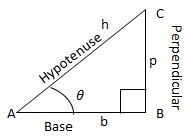• Thus the trigonometric Ratios are defined as the largest side of a right angle triangle is known as hypotenuse.

Also, by Pythagoras theorem, we know that h2 = b+ p2.
Here, θ is pronounced as theta, ∠A=θ. We write trigonometric ratios according to angle θ.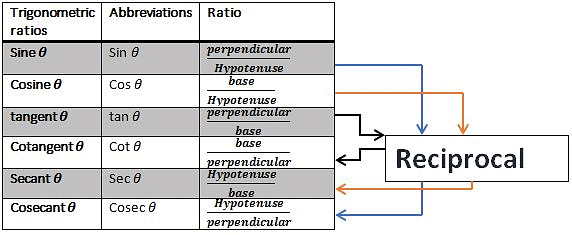Question for Chapter Notes: Introduction to Trigonometry
Try yourself:In a right-angled triangle ABC, the angle ∠A is denoted as θ. Which of the following correctly represents the trigonometric ratio for the angle θ?

## Relation between Trigonometric Ratios

• cosec θ =1/sin θ
• sec θ = 1/cos θ
• tan θ = sin θ/cos θ
• cot θ = cos θ/sin θ=1/tan θ

## Trigonometric Ratios of Specific Angles

### Trigonometric Ratios of 45°

If one of the angles of a right-angled triangle is 45°, then another angle will also be equal to 45°.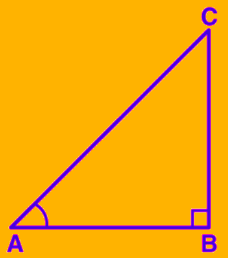Let us say ABC is a right-angled triangle at B, such that;
∠ A = ∠ C = 45°
Thus, BC = AB = a (say)
Using Pythagoras theorem, we have;
AC2 = AB2 + BC2
= a2 + a2
= 2a2
AC = a√2

Now, from the trigonometric ratios, we have;
sin 45° = (Opp. side to angle 45°)/Hypotenuse = BC/AC = a/a√2 = 1/√2
cos 45° = (Adj. side to angle 45°)/Hypotenuse = AB/AC = a/a√2 = 1/√2
tan 45° = BC/AB = a/a = 1

Similarly,
cosec 45° = 1/sin 45° = √2
sec 45° = 1/cos 45° = √2
cot 45° = 1/tan 45° = 1

### Trigonometric Ratios of 30° and 60°

Here, we will consider an equilateral triangle ABC, such that;
AB = BC = AC = 2a
∠A = ∠B = ∠C = 60°
Now, draw a perpendicular AD from vertex A that meets BC at D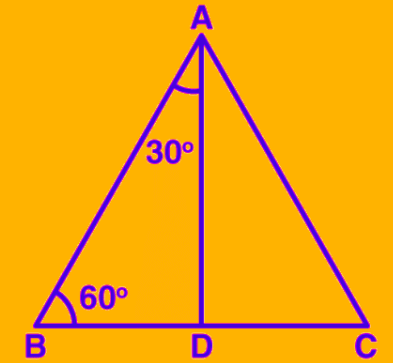According to the congruency of the triangle, we can say;
Δ ABD ≅ Δ ACD
Hence,
BD = DC
Now, in triangle ABD, ∠ BAD = 30° and ∠ ABD = 60°
Using Pythagoras theorem
= (2a)2 – (a)2
= 3a

So, the trigonometric ratios for a 30-degree angle will be;
sin 30° = BD/AB = a/2a = 1/2
cos 30° = AD/AB = a√3/2a = √3/2
tan 30° = BD/AD = a/a√3 = 1/√3

Also,
cosec 30° = 1/sin 30 = 2
sec 30° = 1/cos 30 = 2/√3
cot 30° = 1/tan 30 = √3

Similarly, we can derive the values of trigonometric ratios for 60°.
sin 60° = √3/2
cos 60° = 1/2
tan 60° = √3
cosec 60° = 2/√3
sec 60° = 2
cot 60° = 1/√3

### Trigonometric Ratios of 0° and 90°

If ABC is a right-angled triangle at B, if ∠A is reduced, then side AC will come near to side AB. So, if ∠ A is nearing 0 degree, then AC becomes almost equal to AB, and BC gets almost equal to 0.

Hence, Sin A = BC /AC = 0
and cos A = AB/AC = 1

tan A = sin A/cos A = 0/1 = 0

Also,
cosec A = 1/sin A = 1/0 = not defined
sec A = 1/cos A = 1/1 = 1
cot A = 1/tan A = 1/0 = not defined

In the same way, we can find the values of trigonometric ratios for a 90-degree angle. Here, angle C is reduced to 0, and the side AB will be nearing side BC such that angle A is almost 90 degrees and AB is almost 0.

## Standard Values of Trigonometric Ratios

###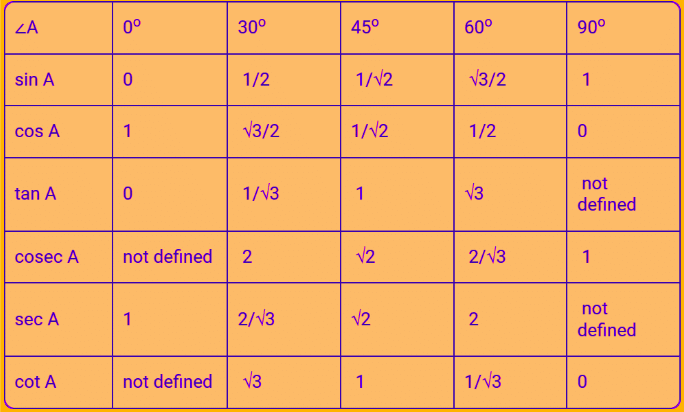Example 1: Evaluate 2 tan2 45° + cos2 30° – sin2 60°.

Solution: Since we know,
tan 45° = 1
cos 30° = √3/2
sin 60° = √3/2
Therefore, putting these values in the given equation:
2(1)2 + (√3/2)2 – (√3/2)2
= 2 + 0
= 2

Question for Chapter Notes: Introduction to Trigonometry
Try yourself:(Sin 30°+cos 60°)-(sin 60° + cos 30°) is equal to:

Example 2: If tan (A + B) =√3 and tan (A – B) =1/√3, 0° < A + B ≤ 90°; A > B, find A and B.

Solution: Given,
tan (A + B) = √3
As we know, tan 60° = √3
Thus, we can write;
⇒ tan (A + B) = tan 60°
⇒(A + B) = 60° …… (i)
Now again given;
tan (A – B) = 1/√3
Since, tan 30° = 1/√3
Thus, we can write;
⇒ tan (A – B) = tan 30°
⇒(A – B) = 30° ….. (ii)
Adding the equation (i) and (ii), we get;
A + B + A – B = 60° + 30°
2A = 90°
A= 45°
Now, put the value of A in eq. (i) to find the value of B;
45° + B = 60°
B = 60° – 45°
B = 15°
Therefore A = 45° and B = 15°

Example 3: Show that :
(i) tan 48° tan 23° tan 42° tan 67° = 1

(ii) cos 38° cos 52° – sin 38° sin 52° = 0

Solution:
(i) tan 48° tan 23° tan 42° tan 67°
We can also write the above given tan functions in terms of cot functions, such as;
tan 48° = tan (90° – 42°) = cot 42°
tan 23° = tan (90° – 67°) = cot 67°
Hence, substituting these values, we get
= cot 42° cot 67° tan 42° tan 67°
= (cot 42° tan 42°) (cot 67° tan 67°)
= 1 × 1 [since cot A.tan A = 1]
= 1
(ii) cos 38° cos 52° – sin 38° sin 52°
We can also write the given cos functions in terms of sin functions.
cos 38° = cos (90° – 52°) = sin 52°
cos 52°= cos (90° – 38°) = sin 38°
Hence, putting these values in the given equation, we get;
sin 52° sin 38° – sin 38° sin 52° = 0

Example 4: If tan 2A = cot (A – 18°), where 2A is an acute angle, find the value of A.

Solution: Given,
tan 2A = cot (A – 18°)
As we know by trigonometric identities,
tan 2A = cot (90° – 2A)
Substituting the above equation in the given equation, we get;
⇒ cot (90° – 2A) = cot (A – 18°)
Therefore,
⇒ 90° – 2A = A – 18°
⇒ 108° = 3A
A = 108° / 3
Hence, the value of A = 36°

Example 5:  If A, B and C are interior angles of a triangle ABC, then show that sin [(B + C)/2] = cos A/2.

Solution:
As we know, for any given triangle, the sum of all its interior angles is equals to 180°.
Thus,
A + B + C = 180° ….(1)
Now we can write the above equation as;
⇒ B + C = 180° – A
Dividing by 2 on both the sides;
⇒ (B + C)/2 = (180° – A)/2
⇒ (B + C)/2 = 90° – A/2
Now, put sin function on both sides.
⇒ sin (B + C)/2 = sin (90° – A/2)
Since,
sin (90° – A/2) = cos A/2
Therefore,
sin (B + C)/2 = cos A/2

## Trigonometric Identities

Identities are equations which are valid for any conditions. Trigonometric identities are equations involving trigonometric ratios.

Some Trigonometric Identities are:

(i) sin2θ + cos2⁡θ = 1

(ii) sec2θ - tan2θ =1

(iii) cosec2θ - cot2⁡θ = 1

Question for Chapter Notes: Introduction to Trigonometry
Try yourself:If cos X = ⅔ then tan X is equal to:

Example 6: Prove the identities:
(i) √[1 + sinA/1 – sinA] = sec A + tan A

(ii) (1 + tan2A/1 + cot2A) = (1 – tan A/1 – cot A)2 = tan2A

Solution:
(i) Given:√[1 + sinA/1 – sinA] = sec A + tan A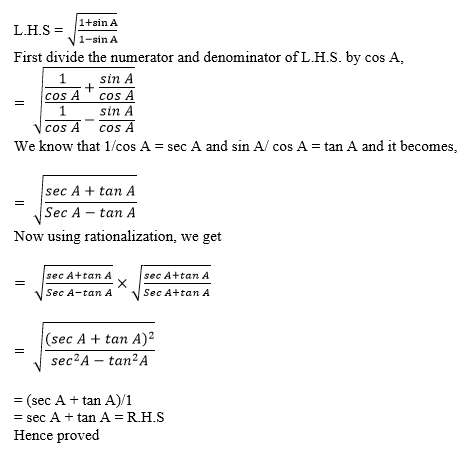(ii) Given: (1 + tan2A/1 + cot2A) = (1 – tan A/1 – cot A)2 = tan2A
LHS:
= (1+tan²A) / (1+cot²A)
Using the trigonometric identities we know that 1+tan²A = sec²A and 1+cot²A= cosec²A
= sec²A/ cosec²A
On taking the reciprocals we get

= sin²A/cos²A

= tan²A

RHS:

=(1-tanA)²/(1-cotA)²

Substituting the reciprocal value of tan A and cot A we get,

= (1-sinA/cosA)²/(1-cosA/sinA)²

= [(cosA-sinA)/cosA]²/ [(sinA-cos)/sinA)²] = [(cosA-sinA)²×sin²A] /[cos²A. /(sinA-cosA)²] =  sin²A/cos²A

= tan2A

The values of LHS and RHS are the same.

Hence proved.

Example 7: If sin θ + cos θ = √3, then prove that tan θ + cot θ = 1.

Solution:

Given,

sin θ + cos θ = √3

Squaring on both sides,

(sin θ + cos θ)= (√3)2

sin2θ + cos2θ + 2 sin θ cos θ = 3

Using the identity sin2A + cos2A = 1,

1 + 2 sin θ cos θ = 3

2 sin θ cos θ = 3 – 1

2 sin θ cos θ = 2

sin θ cos θ = 1

sin θ cos θ = sin2θ + cos2θ

⇒ (sin2θ + cos2θ)/(sin θ cos θ) = 1

⇒ [sin2θ/(sin θ cos θ)] + [cos2θ/(sin θ cos θ)] = 1

⇒ (sin θ/cos θ) + (cos θ/sin θ) = 1

⇒ tan θ + cot θ = 1

Hence proved.

Example 8: Express cot 85° + cos 75° in terms of trigonometric ratios of angles between 0° and 45°.

Solution:

cot 85° + cos 75°

= cot (90° – 5°) + cos (90° – 15°)

We know that cos(90° – A) = sin A and cot(90° – A) = tan A

= tan 5° + sin 15°

## Trigonometric Ratios for Complementary Angles (Deleted from NCERT)

(i) sin (90 – θ) = cosθ

(ii) cos (90 – θ) = sinθ

(iii) tan (90 – θ) = cotθ

(iv) cot (90 – θ) = tanθ

(v) sec (90 – θ) = cosecθ

(vi) cosec (90 – θ) = secθ

The document Introduction to Trigonometry Chapter Notes | Mathematics (Maths) Class 10 is a part of the Class 10 Course Mathematics (Maths) Class 10.
All you need of Class 10 at this link: Class 10

## Mathematics (Maths) Class 10

115 videos|479 docs|129 tests

## Mathematics (Maths) Class 10

115 videos|479 docs|129 testsExplore Courses for Class 10 examSignup to see your scores go up within 7 days! Learn & Practice with 1000+ FREE Notes, Videos & Tests.
10M+ students study on EduRev
Track your progress, build streaks, highlight & save important lessons and more!
Related Searches

,

,

,

,

,

,

,

,

,

,

,

,

,

,

,

,

,

,

,

,

,

;